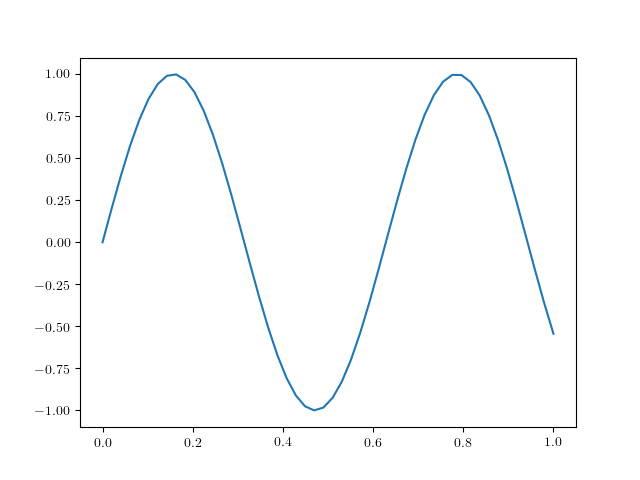# Equation 1: The Heat Equation¶

The heat equation is one of the canonical partial differential equationss with which numericists deal, and an example of a parabolic PDE. As you probably know, the equation for a parabola is

$$x = a y^2.$$

Similar to this, the standard heat equation in one dimension is

$$\frac{\partial T}{\partial t} = \kappa \frac{\partial ^2 T}{\partial t}.$$

This is a model for the temperature of an insulated bar of material, where the flow of heat along the bar is proportional to the local gradient in the bar temperature, moving from high to low temperature. The constant of proportionality, $\kappa$ is called the conductivity in the case of temperature (or generally, the diffusivity) and has units of $m^2/s$.

In :
%matplotlib notebook
import numpy
import pylab
x=numpy.linspace(0,1)
pylab.plot(x,numpy.sin(10*x));In [ ]: